Download Presentation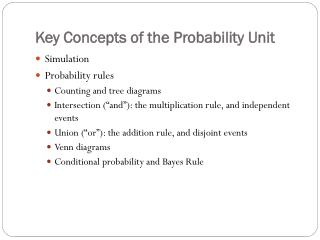Key Concepts of the Probability Unit

# Key Concepts of the Probability Unit - PowerPoint PPT Presentation

Key Concepts of the Probability Unit. Simulation Probability rules Counting and tree diagrams Intersection (“and”): the multiplication rule, and independent events Union (“or”): the addition rule, and disjoint events Venn diagrams Conditional probability and Bayes Rule. Simulation.I am the owner, or an agent authorized to act on behalf of the owner, of the copyrighted work described.
Download Presentation## Key Concepts of the Probability Unit

An Image/Link below is provided (as is) to download presentation

Download Policy: Content on the Website is provided to you AS IS for your information and personal use and may not be sold / licensed / shared on other websites without getting consent from its author.While downloading, if for some reason you are not able to download a presentation, the publisher may have deleted the file from their server.

- - - - - - - - - - - - - - - - - - - - - - - - - - E N D - - - - - - - - - - - - - - - - - - - - - - - - - -
Presentation Transcript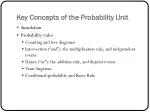Key Concepts of the Probability Unit
• Simulation
• Probability rules
• Counting and tree diagrams
• Intersection (“and”): the multiplication rule, and independent events
• Union (“or”): the addition rule, and disjoint events
• Venn diagrams
• Conditional probability and Bayes Rule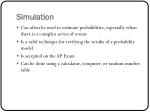Simulation
• Can often be used to estimate probabilities, especially when there is a complex series of events
• Is a valid technique for verifying the results of a probability model
• Is accepted on the AP Exam
• Can be done using a calculator, computer, or random number table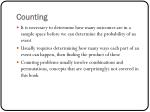Counting
• It is necessary to determine how many outcomes are in a sample space before we can determine the probability of an event
• Usually requires determining how many ways each part of an event can happen, then finding the product of these
• Counting problems usually involve combinations and permutations, concepts that are (surprisingly) not covered in this book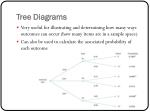Tree Diagrams
• Very useful for illustrating and determining how many ways outcomes can occur (how many items are in a sample space)
• Can also be used to calculate the associated probability of each outcome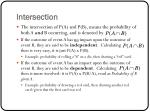Intersection
• The intersection of P(A) and P(B), means the probability of both A and B occurring, and is denoted by
• If the outcome of event A has no impact upon the outcome of event B, they are said to be independent. Calculating then is very easy, it is just P(A) x P(B).
• Example: probability of rolling a “6” on a die, then drawing a “red” card.
• If the outcome of event A has an impact upon the outcome of event B, they are said to be dependent. Calculating then is more involved: it is P(A) x P(B/A), read as Probability of B given A.
• Example: probability of drawing a red card, then drawing another red card/given that the first card was red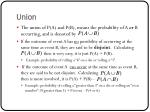Union
• The union of P(A) and P(B), means the probability of A or B occurring, and is denoted by
• If the outcome of event A has no possibility of occurring at the same time as event B, they are said to be disjoint. Calculating then is very easy, it is just P(A) + P(B).
• Example: probability of rolling a “6” on a die or rolling a “3”.
• If the outcome of event A can occur at the same time as event B, they are said to be not disjoint. Calculating then is more involved, it is P(A) + P(B) –
• Example: probability of rolling a “greater than 3” on a die or rolling an “even number”: P(greater than 3) + P(even) – P(4 or 6)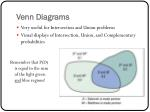Venn Diagrams
• Very useful for Intersection and Union problems
• Visual displays of Intersection, Union, and Complementary probabilities
• Re
• Remember that P(D) is equal to the sum of the light green and blue regions!
• P(D) is equal to the sum of the light green and blue regions!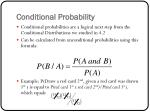Conditional Probability
• Conditional probabilities are a logical next step from the Conditional Distributions we studied in 4.2
• Can be calculated from unconditional probabilities using this formula:
• Example: P(Draw a red card 2nd, given a red card was drawn 1st ) is equal to P(red card 1st x red card 2nd)/P(red card 1st), which equals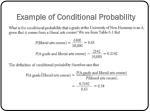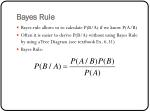Bayes Rule
• Bayes rule allows us to calculate P(B/A) if we know P(A/B)
• Often it is easier to derive P(B/A) without using Bayes Rule by using a Tree Diagram (see textbook Ex. 6.31)
• Bayes Rule:Example of Bayes Rule
• From our previous example, we saw that P(“A”/liberal arts) was 34%. Can we use the information we have to find P(liberal arts/“A”)? Recall that…
• So, P(lib arts/A) = P(A/lib arts)P(lib arts)
• P(A)

(.34)(.63)/.34 = .6314